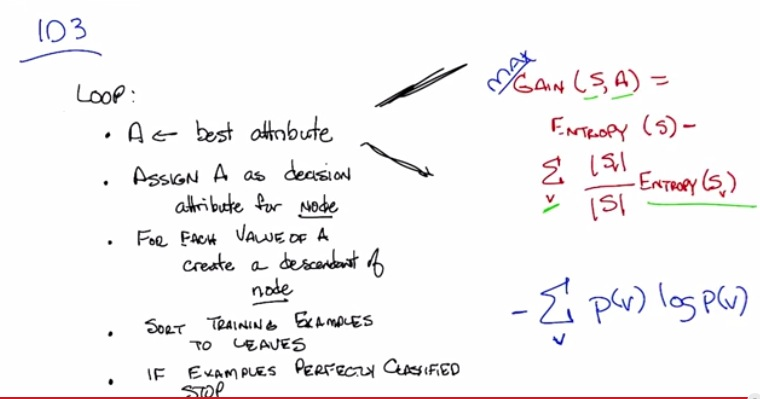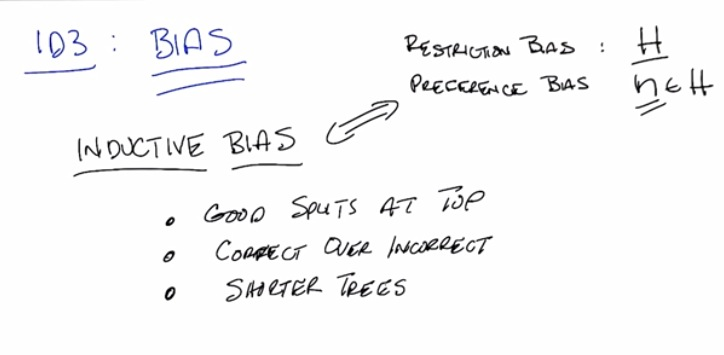• This is the ID3, algorithm to build decision tree model
• First assign A as decision atribute of node
• For each value of A, create descendant of another node.
• Sort training examples to leaves
• If perfectly classified, choose Stop
• Now The red equation is how we use gain function to pick the best attribute
• Blue line is when define the entropy function, which means sum of probability that we have seen the value, times log of probability that we have seen the value.
• When it's fair coin, to decide whether tail or head, we don't know the probability. Probably maybe half. Then we don't know for sure the information. As we flip the coin, for the first time, then the the gain will be at maximum, first gaining information.
• When it's one sided coin, 2 sided head, even before we flip the coin, we know it's going to be head. If we flip it, we don't gain information from it, because we already know. So the gain will be at minimum zero.
• The S is a number of elements our our training example
• and v is notation of value of particular attribute.
• With three examples earlier. First and two example, is the same gain value, at maximum. As we split it, the result of split (both x and o) is still random, we don't get insight at all. Because of that the gain value at maximum
• On the other hand, the final one, split it become perfect as it split both side of x and o. The split makes the result doesn't random at all. And because of that, we don't need to try to gain information again. This makes the gain value at minimum.• Restriction Bias is all the hypothesis to make a decision tree over all the function out there that build the model. This is important as we only care about the only function that build the decision tree.
• Preference Bias is the model that we prefer to build our decision tree, over all the model out there that build the decision tree.
• What we prefer, a good split roughly in half. This is important as we want best split at the top, and more and more to the bottom, taking sort of best split down to the leaves.
• We also want correct, as it as important. In three example earlier, although first one maybe best split in half, but it's incorrect as it doesn't do proper split (both x and o). While the third one, when splitting in half, split perfectly x and o, the most correct one.
• And we also may prefer shorter tree. We may not include all the attributes to make our decision. 2nd example shows how we don't need that attribute to split our data.